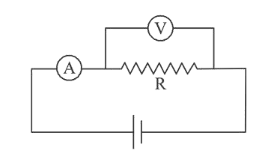# The actual value of resistance R,

Question:

The actual value of resistance $R$, shown in the figure is $30 \Omega$. This is measured in an experiment as shown using

the standard formula $R=\frac{V}{I}$, where $V$ and $I$ are the

reading of the voltmeter and ammeter, respectively. If the measured value of $\mathrm{R}$ is $5 \%$ less, then the internal resistance of the voltmeter is:1. (1) $600 \Omega$

2. (2) $570 \Omega$

3. (3) $35 \Omega$

4. (4) $350 \Omega$

Correct Option: , 2

Solution:

(2) using, $\frac{1}{\mathrm{R}_{\mathrm{eq}}}=\frac{1}{\mathrm{R}_{1}}+\frac{1}{\mathrm{R}_{2}}$

$0.95 \mathrm{R}=\frac{\mathrm{RRv}}{\mathrm{R}+\mathrm{Rv}}$ (measured value $5 \%$ less

then internal resistance of voltmeter)

or, $0.95 \times 30=0.05 \mathrm{Rv}$

$\therefore R v=19 \times 30=570 \Omega$